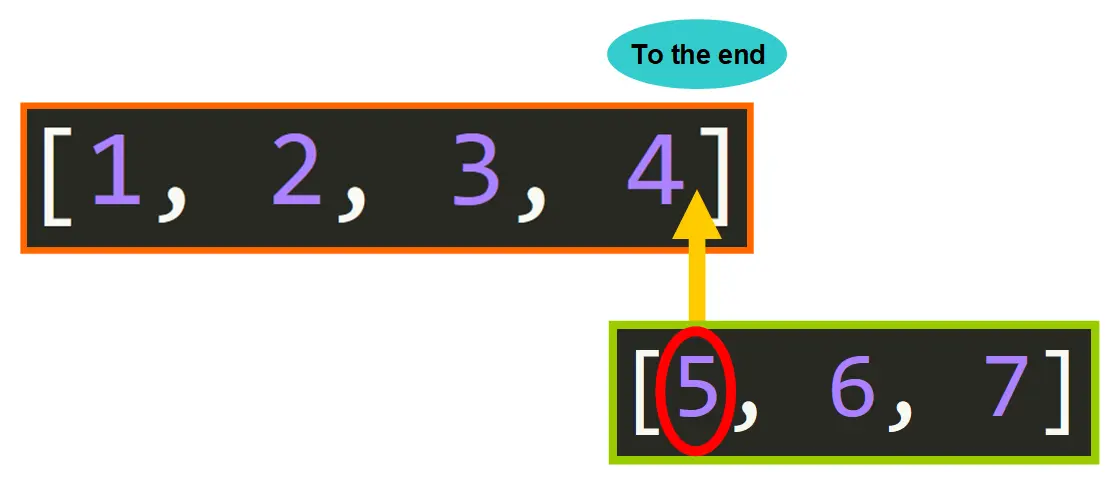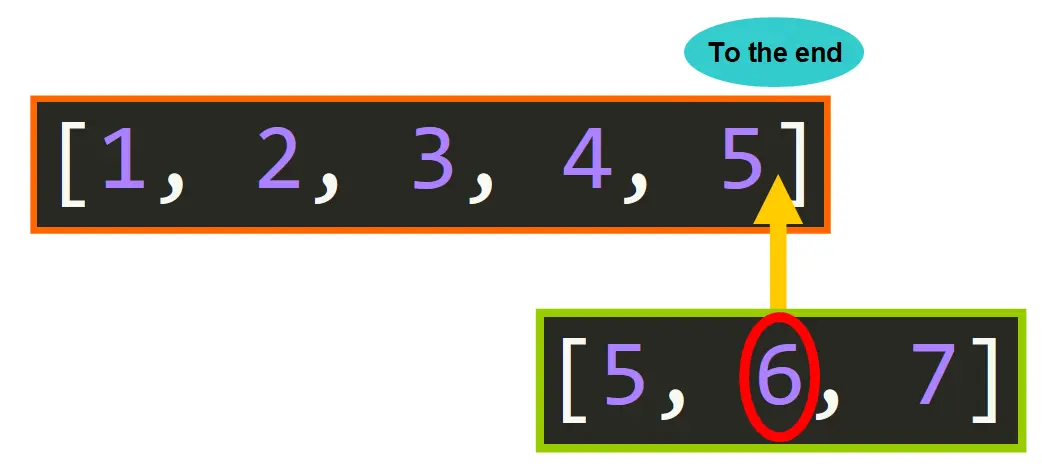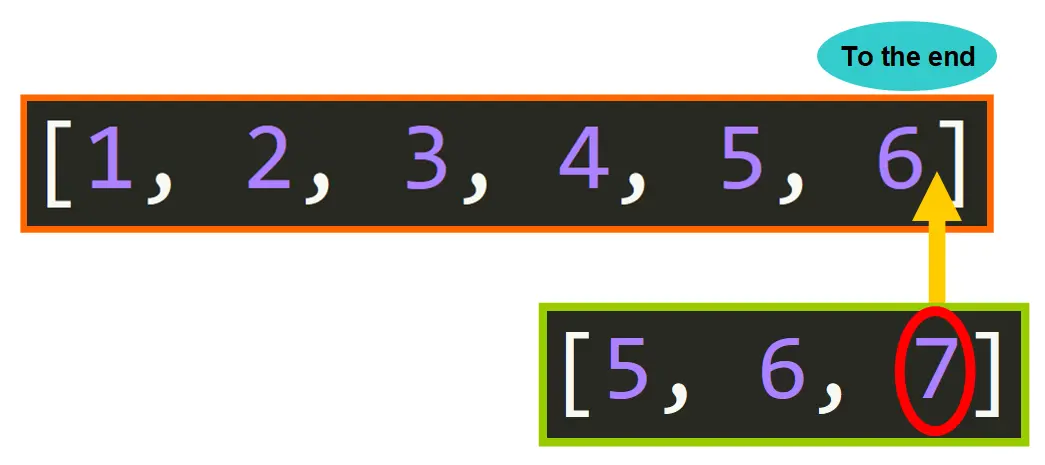# Python List append()

Let's see how the append() method works behind the scenes.

You should use this method when you want to add a single item to the end of a list.python list append()

## Syntax and Arguments

To call the append() method, you will need to use this syntax:

From Left to Right:

• The list that will be modified. This is usually a variable that references a list

• A dot, followed by the name of the method append()

• Within parentheses, the item that will be added to the end of the list

## Examples

Here's an example of how to use list.append():

``````# Define the list
>>> nums = [1, 2, 3, 4]

# Add the integer 5 to the end of the existing list
>>> nums.append(5)

# See the updated value of the list
>>> nums
[1, 2, 3, 4, 5]
``````

Let's pretend that we are conducting a research and that we want to analyze the data collected using Python. We need to add a new measurement to the existing list of values.

How do we do it? We use the list.append() method!

``````# Existing list
>>> nums = [5.6, 7.44, 6.75, 4.56, 2.3]

# Add the float (decimal number) to the end of the existing list
>>> nums.append(7.34)

# See the updated value of the list
>>> nums
[5.6, 7.44, 6.75, 4.56, 2.3, 7.34]
``````

If you are familiar with string, list, or tuple slicing, what append() really does behind the scenes is equivalent to:

``````a[len(a):] = [x]
``````

With this example, you can see that they are equivalent:

Using append()

``````>>> nums = [5.6, 7.44, 6.75, 4.56, 2.3]
>>> nums.append(4.52)
>>> nums
[5.6, 7.44, 6.75, 4.56, 2.3, 4.52]
``````

Using list slicing

``````>>> nums = [5.6, 7.44, 6.75, 4.56, 2.3]
>>> nums[len(nums):] = [4.52]
>>> nums
[5.6, 7.44, 6.75, 4.56, 2.3, 4.52]
``````

### Appending a Sequence

``````>>> nums = [5.6, 7.44, 6.75, 4.56, 2.3]
>>> nums.append([5.67, 7.67, 3.44])
>>> nums
# OUTPUT?
``````

Are you ready? This will be the output:

``````[5.6, 7.44, 6.75, 4.56, 2.3, [5.67, 7.67, 3.44]]
``````

You might be asking, why was the full list added as a single item? It's because the append() method adds the entire item to the end of the list. If the item is a sequence such as a list, dictionary, or tuple, the entire sequence will be added as a single item of the existing list.

Here we have another example (below). In this case, the item is a tuple and it is added as a single item of the list, not as individual items:

``````>>> names = ["Lulu", "Nora", "Gino", "Bryan"]
>>> names.append(("Emily", "John"))
>>> names
['Lulu', 'Nora', 'Gino', 'Bryan', ('Emily', 'John')]
``````

# Extend

Now let's dive into the functionality of the extend() method.

You should use this method if you need to append several items to a list as individual items.

Let me illustrate the importance of this method with a familiar friend that you just learned: the append() method. Based on what you've learned so far, if we wanted to add several individual items to a list using append(), we would need to use append() several times, like this:

``````# List that we want to modify
>>> nums = [5.6, 7.44, 6.75, 4.56, 2.3]

# Appending the items
>>> nums.append(2.3)
>>> nums.append(9.6)
>>> nums.append(4.564)
>>> nums.append(7.56)

# Updated list
>>> nums
[5.6, 7.44, 6.75, 4.56, 2.3, 2.3, 9.6, 4.564, 7.56]
``````

I'm sure that you are probably thinking that this would not be very efficient, right? What if I need to add thousands or millions of values? I cannot write thousands or millions of lines for this simple task. That would take forever!

So let's see an alternative. We can store the values that we want to add in a separate list and then use a for loop to call append() as many times as needed:

``````# List that we want to modify
>>> nums = [5.6, 7.44, 6.75, 4.56, 2.3]

# Values that we want to add
>>> new_values = [2.3, 9.6, 4.564, 7.56]

# For loop that is going to append the value
>>> for num in new_values:
nums.append(num)

# Updated value of the list
>>> nums
[5.6, 7.44, 6.75, 4.56, 2.3, 2.3, 9.6, 4.564, 7.56]
``````

This is more efficient, right? We are only writing a few lines. But there is an even more efficient, readable, and compact way to achieve the same purpose: list.extend()!

``````>>> nums = [5.6, 7.44, 6.75, 4.56, 2.3]
>>> new_values = [2.3, 9.6, 4.564, 7.56]

# This is where the magic occurs! No more for loops
>>> nums.extend(new_values)

# The list was updated with individual values
>>> nums
[5.6, 7.44, 6.75, 4.56, 2.3, 2.3, 9.6, 4.564, 7.56]
``````

Let's see how this method works behind the scenes.

### Syntax and Arguments

To call the list.extend() method, you will need to use this syntax:

From Left to Right:

• The list that will be modified. This is usually a variable that refers to the list.

• A dot . (So far, everything is exactly the same as before).

• The name of the method extend() (Now things start to change...).

• Within parentheses, an iterable (list, tuple, dictionary, set, or string) that contains the items that will be added as individual elements of the list.

### Behind the Scenes

Let's see how python extend() works behind the scenes. Here we have an example:

``````# List that will be modified
>>> a = [1, 2, 3, 4]

# Sequence of values that we want to add to the list a
>>> b = [5, 6, 7]

# Calling .extend()
>>> a.extend(b)

# See the updated list. Now the list a has the values 5, 6, and 7
>>> a
[1, 2, 3, 4, 5, 6, 7]
``````

You can think of list.extend() as a method that appends the individual elements of the iterable in the same order as they appear.

In this case, we have a list a = [1, 2, 3, 4] as illustrated in the diagram below. We also have a list b = [5, 6, 7] that contains the sequence of values that we want to add. The method takes each element of b and appends it to list a in the same order.First element is appended.Second element appended.Third element appended.

After this process is completed, we have the updated list a and we can work with the values as individual elements of a.

### Examples

You may be curious to know how the python list.extend() method works when you pass different types of iterables. Let's see how in the following examples:

#### Tuples

The process works exactly the same if you pass a tuple. The individual elements of the tuple are appended one by one in the order that they appear.

``````# List that will be extended
>>> a = [1, 2, 3, 4]

# Values that will be added (the iterable is a tuple!)
>>> b = (1, 2, 3, 4)

# Method call
>>> a.extend(b)

# The value of the list a was updated
>>> a
[1, 2, 3, 4, 1, 2, 3, 4]
``````

#### Sets

The same occurs if you pass a set. The elements of the set are appended one by one.

``````# List that will be extended
>>> a = [1, 2, 3, 4]

# Values that will be appended (the iterable is a set!)
>>> c = {5, 6, 7}

# Method call
>>> a.extend(c)

# The value of a was updated
>>> a
[1, 2, 3, 4, 5, 6, 7]
``````

#### Strings

Strings work a little bit different with the list.extend() method. Each character of the string is considered an "item", so the characters are appended one by one in the order that they appear in the string.

``````# List that will be extended
>>> a = ["a", "b", "c"]

# String that will be used to extend the list
>>> b = "Hello, World!"

# Method call
>>> a.extend(b)

# The value of a was updated
>>> a
['a', 'b', 'c', 'H', 'e', 'l', 'l', 'o', ',', ' ', 'W', 'o', 'r', 'l', 'd', '!']
``````

#### Dictionaries

Dictionaries have a particular behavior when you pass them as arguments to list.extend(). In this case, the dictionary keys of the dictionary are appended one by one. The values of the corresponding key-value pairs are not appended to the list a.

``````# List that will be extended
>>> a = ["a", "b", "c"]

# Dictionary that will be used to extend the list
>>> b = {"d": 5, "e": 6, "f": 7}

# Method call
>>> a.extend(b)

# The value of a was updated
>>> a
['a', 'b', 'c', 'd', 'e', 'f']
``````

### Equivalent to...

What list.extend() does is equivalent to a[len(a):] = iterable. Here we have an example to illustrate that they are equivalent:

**Using list.extend():

``````# List that will be extended
>>> a = [1, 2, 3, 4]

# Values that will be appended
>>> b = (6, 7, 8)

# Method call
>>> a.extend(b)

# The list was updated
>>> a
[1, 2, 3, 4, 6, 7, 8]
``````

Using list slicing:

``````# List that will be extended
>>> a = [1, 2, 3, 4]

# Values that will be appended
>>> b = (6, 7, 8)

# Assignment statement. Assign the iterable b as the final portion of the list a
>>> a[len(a):] = b

# The value of a was updated
>>> a
[1, 2, 3, 4, 6, 7, 8]
``````

The result is the same, but using list.extend() is much more readable and compact, right? Python truly offers amazing tools to improve our workflow.

# Summary of their Differences

Now that you know how to work with list.append() and list.extend(), let's see a summary of their key differences:

• list.append() adds a single element to the end of the list while list.extend() can add multiple individual elements to the end of the list.

• list.append() takes a single element as argument while list.extend() takes an iterable as argument (list, tuple, dictionaries, sets, strings).

In this example (below), the keys are "d", "e", and "f". These values are appended to the list a.

# SUBSCRIBE FOR NEW ARTICLES